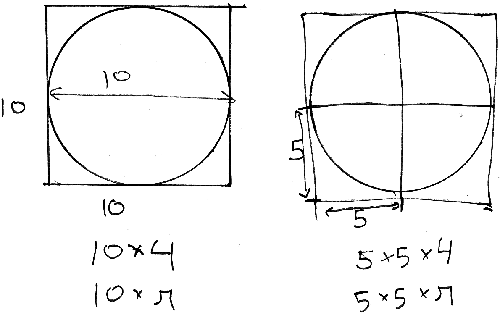# Circle circumference and area insight

Written March 5, 2008. Tagged Geometry.

I wanted to share an insight I had not many years ago. Perhaps this is obvious to everyone else; I've no idea.

So the circumference of a circle is `diameter × π`; the area is `radius × radius × π`.

I sometimes forgot what the calculations were exactly, since I'd just learned them by rote without really knowing the why of it.

Sometime not too long ago I suddenly had an insight on the subject; I haven't forgotten them since. The insight is this:Calculating the circumference and area of a square is a lot more intuitive. So let's put the circle inside a square. Though my sloppy drawing suggests otherwise, the square is snug against the circle. The circle and square share the same diameter and radius, if you'll allow using those terms for a square. (It only shares the "radius" from the center and straight up, down, left or right, of course.)

Now, just as the circumference of the square is `diameter × 4`, the circumference of the circle is `diameter × π`.

Just as the area of the square can be described as `radius × radius × 4` (split into four squares and sum the areas), the area of the circle is `radius × radius × π`.

So the calculations are the same, except for this: in these matters, a circle is to a square as π is to 4. So the magic number ~3.14 means in some sense that every circle is ~0.86 short of a square.

Again, this might be obvious to everyone else since forever. I'm sure I also said things that will offend math geeks. Do correct any transgressions. I found it an interesting insight and a great mnemonic.